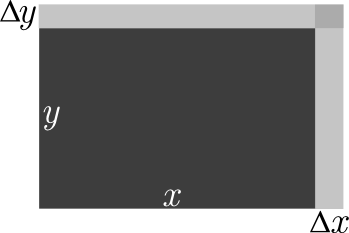## The Product Rule

In calculus textbooks, the product rule for differentiation is often presented as a theorem. The authors then prove the theorem using the definition of the derivative. Other authors choose to simply write out the steps of the proof and present the product rule as a useful identity without motivating the mathematical steps (see MathWorld for an example). For those of us who prefer to think visually, both of these approaches are somewhat unsatisfactory.  On this page, I present the product rule in a more visual / geometric way.  The explanation presented below may not be considered “rigorous” enough for a mathematician, however it’s a suitable explanation for a physicist.Suppose the product we are differentiating is the area of a rectangle, $$A$$. If the width and length of the rectangle are $$x$$ and $$y$$, then $$!A=xy$$ Suppose the dimensions of the rectangle can change with time so that $$x = x(t)$$, $$y = y(t)$$, and $$A = A(t)$$.  Further suppose that, in a time interval $$\Delta t$$, the area of the rectangle changes by $$\Delta A$$. $$!\Delta A = x\Delta y+y\Delta x+\Delta x\Delta y$$ The average rate of change of the area with respect to time is then $$!\displaystyle\frac{\Delta A}{\Delta t}=x\frac{\Delta y}{\Delta t}+y\frac{\Delta x}{\Delta t}+\frac{\Delta x\Delta y}{\Delta t}$$ In the limit as $$\Delta t\rightarrow0$$, we see that $$\Delta A$$, $$\Delta x$$, and $$\Delta y$$ also approach zero. In fact,  if $$x$$, $$y$$, and $$A$$ are continuous functions of $$t$$: $$!\displaystyle\lim_{\Delta t\rightarrow0}\frac{\Delta A}{\Delta t} = \frac{{\rm d}A}{{\rm d}t}$$ $$!\displaystyle\lim_{\Delta t\rightarrow0}\frac{\Delta x}{\Delta t} = \frac{{\rm d}x}{{\rm d}t}$$ $$!\displaystyle\lim_{\Delta t\rightarrow0}\frac{\Delta y}{\Delta t} = \frac{{\rm d}y}{{\rm d}t}$$ The average rates of change become derivatives.  Thus, the derivative of the product $$x(t)y(t)$$ with respect to $$t$$ is $$!\displaystyle\frac{{\rm d}}{{\rm d}t}\left[x(t)y(t)\right]=\frac{{\rm d}A}{{\rm d}t}=\lim_{\Delta t\rightarrow0}\left[ y\frac{\Delta x}{\Delta t}+x\frac{\Delta y}{\Delta t} +\frac{\Delta x\Delta y}{\Delta t}\right]$$ or simply $$!\displaystyle\frac{{\rm d}}{{\rm d}t}\left[x(t)y(t)\right]=y\frac{{\rm d}x}{{\rm d}t}+x\frac{{\rm d} y}{{\rm d}t}$$ Which is what we all know as the “product rule” for differentiation.